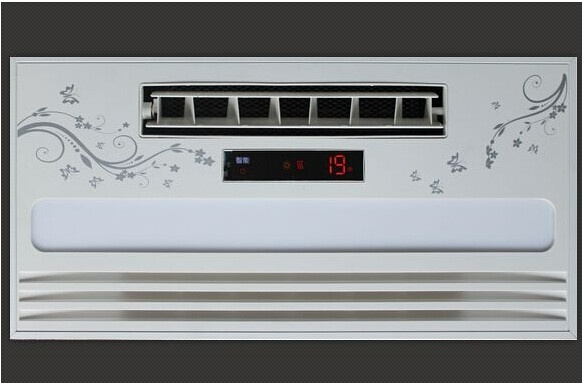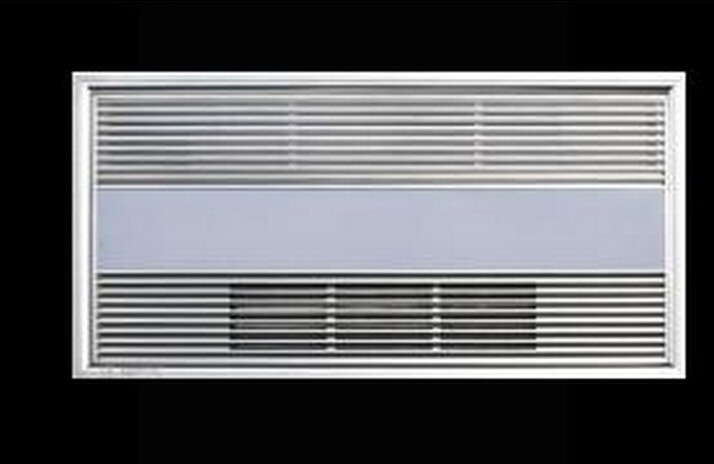|

# 四类冬季采暖方式优缺点pk 空调电暖气壁挂炉地暖

空调、电暖气、壁挂炉等取暖设备纷纷登场，到底这些取暖器材都各有哪些优缺点?哪种最适合您家?不妨听听结合业内人士的说法。采暖方式推荐一、可控性强但很费电

费用：5000元左右;取暖性：★★★★;舒适性：★★★

种类：在很多没有集中供暖的家庭，最常用来替代集中供暖的，就是用空调取暖了。因为购买空调的成本相对低，可控性较强，很受人们欢迎，按照安装情况等的不同，用于冬季取暖的空调有中央空调、普通冷暖空调以及可移动空调等几种。

优点：总的来说，不管是哪种空调取暖方式，最大的优点就是操作简单方便，启动升温速度快，且能够定时、定温，精确调节温度。此外，不用的时候，可以将之关闭，非常灵活。而且对于集中供暖可以起到一个补充作用，很多家中有老人和孩子的家庭，在集中供暖之前、之后，都要开一段时间的空调取暖。这其中最舒适的应该是中央空调，解决了风口直接吹向人的缺点，气流组织好，使得整个房间都处于空调的回风区域，人的感觉最好。

缺点：空调的缺点相信很多人都知道，最大的一个就是太费电。特别是那些家中24小时都有人的家庭，要一直开着空调就会更加费电。空调的另一个缺点就是舒适性较差，除了中央空调之外，普通冷暖空调和可移动的小空调在这方面表现尤为突出，冲着风口的地方很热，不冲风口的地方又很冷，而且开空调会使得整个屋里过于干燥，在冬天感受尤其不好，因此一般使用空调取暖的家庭，都会再配备加湿器使用。另外，由于空调是依靠热风强吹到屋面的中下部，热量分布不均匀，上热下凉。而且空气对流猛烈，机械性强制吹风让人感觉不舒服，且加快空气中的水分蒸发和扬起灰尘，降低了室内空气质量，严重的会诱发呼吸道疾病。

采暖方式推荐二、耗电较高不太安全

费用：500元左右;取暖性：★★★;舒适性：★★

种类：还有很多租房住的年轻人，偏爱电暖器取暖这种方式，特别是在我国南方没有集中供暖，大部分家庭都只能使用电暖器取暖。室内电暖器均属于直接电热产品，能够便捷地通过开停电源来控制电暖器的温度。目前市场上比较常见的电暖器，根据取暖原理不同，分为油汀式、PTC暖风机、对流式、电热膜等几类，加上其功能特点以及功率大小，取暖的效果也各异。在青岛，比较常见的就是油汀式电暖器和小太阳。

优点：电暖器之所以受到很多年轻人的喜爱，主要原因就是成本比较低，比较便宜的电暖器，价格在一百多元到几百元间，不像空调那样动辄几千元，经济实惠，适合租住房子的人群。另外，电暖器的可控性比较强，能够便捷地通过开停电源来控制电暖器的温度，且移动方便。对于寒冬期不长的地区来说，电暖气能够起到一定的保暖作用。

缺点：尽管电暖器便宜，但它的缺点也不少。首先，电暖器的舒适度不高，很多用过电暖器的人都有体会，不管是油汀式的还是小太阳，都是靠近它的地方很热，甚至热得受不了，但离开它的辐射范围，就会觉得特别冷。而且用电暖器取暖会造成屋内干燥、产生静电。运行时温度不均匀，不利于身体健康。特别要提醒的是，电暖器发热元件有烫伤婴幼儿的危险。另外，电暖器的使用寿命也比较短，因为电暖器是靠开关来控制，发热元件长期高温工作，会降低使用寿命，而且电暖器都有一定的辐射，对健康也有不好的影响。另外，如果晚上忘记关小太阳或者纸张等过于靠近小太阳，还容易引起火灾，这些都值得引起重视。采暖方式推荐三、前期投入高 取暖效果好

费用：15000元左右;取暖性：★★★★★;舒适性：★★★★

分类：壁挂炉取暖是近年来比较流行的一种取暖方式，尤其是在北方那些还不能享受集中供暖的家庭，壁挂炉取暖可以说是最接近集中供暖效果，而且又省钱的一种取暖方式。目前市面上比较流行的，安全性和功效性比较高的壁挂炉品牌有菲斯曼壁挂炉和德国威能壁挂炉等。袁胜奇介绍，燃气壁挂炉又称燃气取暖热水炉。以天然气、人工煤气或液化气作为燃料，燃料经燃烧器输出，在燃烧室内燃烧后，由热交换器将热量吸收，取暖系统中的循环水在途经热交换器时，经过往复加热、从而不断将热量输出给建筑物，为建筑物提供热源。目前，常用的壁挂炉分为取暖生活热水两用炉和单纯暖炉两种。

优点：壁挂炉取暖的优点相比其他取暖方式是最多的。首先，壁挂炉取暖的舒适度最高，因为是通过燃气来烧热水供暖，基本和我们使用集中供暖的原理差不多，相当于在家里安了一个烧燃气的小锅炉，所以升温很均匀，人体的感觉最舒适，而且干净卫生。其次，壁挂炉取暖的可控性比较强，每个房间都有开关，待在哪个房间就把哪个房间的开关打开，如果不在家，可以直接把炉子关掉，这样就最大程度地减少了资源的浪费，同时可以省掉不少燃气的费用。壁挂炉取暖还有一个优点就是可以省掉买热水器的费用，因为在用壁挂炉供暖的同时，它还可以为家中提供生活用的热水，等同于一个燃气热水器，所以是一物两用。最后，就是它可以作为集中供暖的一个补充，对有老人小孩等特殊人群的家庭，在供暖前后，可以使用壁挂炉取暖，要比使用空调取暖舒适很多。

缺点：当然，壁挂炉取暖也不是完美的，首先它的安装费用较高。目前市场上的壁挂炉有国产、进口品牌国产和纯进口这几种，价格从不足一万元到几万元不等，一个好的壁挂炉造价相对较高。其次就是燃气的费用可能会比集中供暖略高，以一个建筑面积100平方米的房子来算，如果24小时都开着壁挂炉，燃气的费用可能比集中供暖费高出20%至40%，但由于壁挂炉的可控性较好，大部分家庭使用壁挂炉的费用要比集中供暖费少。最后一个缺点就是壁挂炉一般只适用于有通天然气的社区，且安装最好与装修同步完成，最好在毛坯阶段就完成，否则会存在散热片和热水管外露的问题，影响居室的整体美观性。此外，壁挂炉需要安装在阳台、厨房等通风好的地方，且存在一定的噪音。

采暖方式推荐四、热得更加均匀 舒适性更高

费用：20000元左右;取暖性：★★★★★;舒适性：★★★★

分类：地暖供暖也是近年来比较流行的一种取暖方式，除了集中供暖时会采用到地暖外，很多没法享受集中供暖的人，也会在家里自己安装地暖。目前比较流行的地暖一般可分为水地暖和电地暖两种。水地暖是以温度不高于60℃的热水埋置于地面以下填充层中的加热管内循环流动，加热整个地板，通过地面以辐射和对流的热传递方式向室内供热的一种供暖方式。电地暖是将外表面允许工作温度上限为65℃的发热电缆埋设在地板中，以发热电缆为热源加热地板，以温控器控制室温或地板温度，实现地面辐射供暖的供暖方式。前面介绍的壁挂炉取暖中，就可能包含水地暖。

优点：地暖相对于其他的取暖方式来说，热得更加均匀，舒适性更高 ，因此也越来越受到人们的青睐。下面就来分别说一下水地暖和电地暖的优点。水地暖的安全性和舒适性较高，因为水地暖是在地板下面盘管来进行安装，没有接口，只要是管线合格，一般不存在漏水的问题，使用寿命都在50年以上。电地暖相对来说也较为安全，但是要考虑到能否承受电地暖的大功率，以免产生意外。另外，电地暖最大的优点就是比较灵活，可以在房子装修好之后安装，也可以在某一个房间单独安装，而且电地暖升温很迅速，一般半小时到一小时之内就能升温。

缺点：水地暖的缺点前面已经说到过，就是安装时尽量选在入住之前，房子毛坯阶段进行。水地暖前期的投资较大，需要安装锅炉。电地暖不用安装锅炉，前期投资很小，但是后期用电的费用很高。另外，王俊也提醒大家，在安装水地暖的时候，不要觉得水管盘得越密越好，一定要请专业的人员来进行测量，根据房间的南北朝向、是否有冷墙、窗户的密封性等因素进行设计，这样才能让自己居住起来更加舒适。供暖期注意加强湿度缓解干燥

供暖后，虽然人们感受到了融融暖意，但室内空气湿度却随之下降了，这样一来不但让人感觉不适，也极易诱发各种疾病，如皮肤干燥、皮肤瘙痒等。专家建议，及时采取人工措施加强室内空气湿度，比如用加湿器加湿，也可以在暖气旁边放盆水，或者用几块湿毛巾搭在暖气片上，让水慢慢蒸发，保持室内空气湿润。专家介绍，空气湿度在45%至55%之间比较合适。专家指出，湿度低于40%时，鼻部和肺部呼吸黏膜上的纤毛运动减弱，病毒、细菌极易附在黏膜上，刺激咽喉部引发咳嗽等不适。

`声明：本文由入驻焦点开放平台的作者撰写，除焦点官方账号外，观点仅代表作者本人，不代表焦点立场错误信息举报电话： 400-099-0099，邮箱：jubao@vip.sohu.com，或点此进行意见反馈，或点此进行举报投诉。`A B C D E F G H J K L M N P Q R S T W X Y Z
A - B - C - D - E
• A
• 鞍山
• 安庆
• 安阳
• 安顺
• 安康
• 澳门
• B
• 北京
• 保定
• 包头
• 巴彦淖尔
• 本溪
• 蚌埠
• 亳州
• 滨州
• 北海
• 百色
• 巴中
• 毕节
• 保山
• 宝鸡
• 白银
• 巴州
• C
• 承德
• 沧州
• 长治
• 赤峰
• 朝阳
• 长春
• 常州
• 滁州
• 池州
• 长沙
• 常德
• 郴州
• 潮州
• 崇左
• 重庆
• 成都
• 楚雄
• 昌都
• 慈溪
• 常熟
• D
• 大同
• 大连
• 丹东
• 大庆
• 东营
• 德州
• 东莞
• 德阳
• 达州
• 大理
• 德宏
• 定西
• 儋州
• 东平
• E
• 鄂尔多斯
• 鄂州
• 恩施
F - G - H - I - J
• F
• 抚顺
• 阜新
• 阜阳
• 福州
• 抚州
• 佛山
• 防城港
• G
• 赣州
• 广州
• 桂林
• 贵港
• 广元
• 广安
• 贵阳
• 固原
• H
• 邯郸
• 衡水
• 呼和浩特
• 呼伦贝尔
• 葫芦岛
• 哈尔滨
• 黑河
• 淮安
• 杭州
• 湖州
• 合肥
• 淮南
• 淮北
• 黄山
• 菏泽
• 鹤壁
• 黄石
• 黄冈
• 衡阳
• 怀化
• 惠州
• 河源
• 贺州
• 河池
• 海口
• 红河
• 汉中
• 海东
• 怀来
• I
• J
• 晋中
• 锦州
• 吉林
• 鸡西
• 佳木斯
• 嘉兴
• 金华
• 景德镇
• 九江
• 吉安
• 济南
• 济宁
• 焦作
• 荆门
• 荆州
• 江门
• 揭阳
• 金昌
• 酒泉
• 嘉峪关
K - L - M - N - P
• K
• 开封
• 昆明
• 昆山
• L
• 廊坊
• 临汾
• 辽阳
• 连云港
• 丽水
• 六安
• 龙岩
• 莱芜
• 临沂
• 聊城
• 洛阳
• 漯河
• 娄底
• 柳州
• 来宾
• 泸州
• 乐山
• 六盘水
• 丽江
• 临沧
• 拉萨
• 林芝
• 兰州
• 陇南
• M
• 牡丹江
• 马鞍山
• 茂名
• 梅州
• 绵阳
• 眉山
• N
• 南京
• 南通
• 宁波
• 南平
• 宁德
• 南昌
• 南阳
• 南宁
• 内江
• 南充
• P
• 盘锦
• 莆田
• 平顶山
• 濮阳
• 攀枝花
• 普洱
• 平凉
Q - R - S - T - W
• Q
• 秦皇岛
• 齐齐哈尔
• 衢州
• 泉州
• 青岛
• 清远
• 钦州
• 黔南
• 曲靖
• 庆阳
• R
• 日照
• 日喀则
• S
• 石家庄
• 沈阳
• 双鸭山
• 绥化
• 上海
• 苏州
• 宿迁
• 绍兴
• 宿州
• 三明
• 上饶
• 三门峡
• 商丘
• 十堰
• 随州
• 邵阳
• 韶关
• 深圳
• 汕头
• 汕尾
• 三亚
• 三沙
• 遂宁
• 山南
• 商洛
• 石嘴山
• T
• 天津
• 唐山
• 太原
• 通辽
• 铁岭
• 泰州
• 台州
• 铜陵
• 泰安
• 铜仁
• 铜川
• 天水
• 天门
• W
• 乌海
• 乌兰察布
• 无锡
• 温州
• 芜湖
• 潍坊
• 威海
• 武汉
• 梧州
• 渭南
• 武威
• 吴忠
• 乌鲁木齐
X - Y - Z
• X
• 邢台
• 徐州
• 宣城
• 厦门
• 新乡
• 许昌
• 信阳
• 襄阳
• 孝感
• 咸宁
• 湘潭
• 湘西
• 西双版纳
• 西安
• 咸阳
• 西宁
• 仙桃
• 西昌
• Y
• 运城
• 营口
• 盐城
• 扬州
• 鹰潭
• 宜春
• 烟台
• 宜昌
• 岳阳
• 益阳
• 永州
• 阳江
• 云浮
• 玉林
• 宜宾
• 雅安
• 玉溪
• 延安
• 榆林
• 银川
• Z
• 张家口
• 镇江
• 舟山
• 漳州
• 淄博
• 枣庄
• 郑州
• 周口
• 驻马店
• 株洲
• 张家界
• 珠海
• 湛江
• 肇庆
• 中山
• 自贡
• 资阳
• 遵义
• 昭通
• 张掖
• 中卫

1室1厅1厨1卫1阳台

1
2
3
4
5

0
1
2

1

1

0
1
2
3报名成功，资料已提交审核A B C D E F G H J K L M N P Q R S T W X Y Z
A - B - C - D - E
• A
• 鞍山
• 安庆
• 安阳
• 安顺
• 安康
• 澳门
• B
• 北京
• 保定
• 包头
• 巴彦淖尔
• 本溪
• 蚌埠
• 亳州
• 滨州
• 北海
• 百色
• 巴中
• 毕节
• 保山
• 宝鸡
• 白银
• 巴州
• C
• 承德
• 沧州
• 长治
• 赤峰
• 朝阳
• 长春
• 常州
• 滁州
• 池州
• 长沙
• 常德
• 郴州
• 潮州
• 崇左
• 重庆
• 成都
• 楚雄
• 昌都
• 慈溪
• 常熟
• D
• 大同
• 大连
• 丹东
• 大庆
• 东营
• 德州
• 东莞
• 德阳
• 达州
• 大理
• 德宏
• 定西
• 儋州
• 东平
• E
• 鄂尔多斯
• 鄂州
• 恩施
F - G - H - I - J
• F
• 抚顺
• 阜新
• 阜阳
• 福州
• 抚州
• 佛山
• 防城港
• G
• 赣州
• 广州
• 桂林
• 贵港
• 广元
• 广安
• 贵阳
• 固原
• H
• 邯郸
• 衡水
• 呼和浩特
• 呼伦贝尔
• 葫芦岛
• 哈尔滨
• 黑河
• 淮安
• 杭州
• 湖州
• 合肥
• 淮南
• 淮北
• 黄山
• 菏泽
• 鹤壁
• 黄石
• 黄冈
• 衡阳
• 怀化
• 惠州
• 河源
• 贺州
• 河池
• 海口
• 红河
• 汉中
• 海东
• 怀来
• I
• J
• 晋中
• 锦州
• 吉林
• 鸡西
• 佳木斯
• 嘉兴
• 金华
• 景德镇
• 九江
• 吉安
• 济南
• 济宁
• 焦作
• 荆门
• 荆州
• 江门
• 揭阳
• 金昌
• 酒泉
• 嘉峪关
K - L - M - N - P
• K
• 开封
• 昆明
• 昆山
• L
• 廊坊
• 临汾
• 辽阳
• 连云港
• 丽水
• 六安
• 龙岩
• 莱芜
• 临沂
• 聊城
• 洛阳
• 漯河
• 娄底
• 柳州
• 来宾
• 泸州
• 乐山
• 六盘水
• 丽江
• 临沧
• 拉萨
• 林芝
• 兰州
• 陇南
• M
• 牡丹江
• 马鞍山
• 茂名
• 梅州
• 绵阳
• 眉山
• N
• 南京
• 南通
• 宁波
• 南平
• 宁德
• 南昌
• 南阳
• 南宁
• 内江
• 南充
• P
• 盘锦
• 莆田
• 平顶山
• 濮阳
• 攀枝花
• 普洱
• 平凉
Q - R - S - T - W
• Q
• 秦皇岛
• 齐齐哈尔
• 衢州
• 泉州
• 青岛
• 清远
• 钦州
• 黔南
• 曲靖
• 庆阳
• R
• 日照
• 日喀则
• S
• 石家庄
• 沈阳
• 双鸭山
• 绥化
• 上海
• 苏州
• 宿迁
• 绍兴
• 宿州
• 三明
• 上饶
• 三门峡
• 商丘
• 十堰
• 随州
• 邵阳
• 韶关
• 深圳
• 汕头
• 汕尾
• 三亚
• 三沙
• 遂宁
• 山南
• 商洛
• 石嘴山
• T
• 天津
• 唐山
• 太原
• 通辽
• 铁岭
• 泰州
• 台州
• 铜陵
• 泰安
• 铜仁
• 铜川
• 天水
• 天门
• W
• 乌海
• 乌兰察布
• 无锡
• 温州
• 芜湖
• 潍坊
• 威海
• 武汉
• 梧州
• 渭南
• 武威
• 吴忠
• 乌鲁木齐
X - Y - Z
• X
• 邢台
• 徐州
• 宣城
• 厦门
• 新乡
• 许昌
• 信阳
• 襄阳
• 孝感
• 咸宁
• 湘潭
• 湘西
• 西双版纳
• 西安
• 咸阳
• 西宁
• 仙桃
• 西昌
• Y
• 运城
• 营口
• 盐城
• 扬州
• 鹰潭
• 宜春
• 烟台
• 宜昌
• 岳阳
• 益阳
• 永州
• 阳江
• 云浮
• 玉林
• 宜宾
• 雅安
• 玉溪
• 延安
• 榆林
• 银川
• Z
• 张家口
• 镇江
• 舟山
• 漳州
• 淄博
• 枣庄
• 郑州
• 周口
• 驻马店
• 株洲
• 张家界
• 珠海
• 湛江
• 肇庆
• 中山
• 自贡
• 资阳
• 遵义
• 昭通
• 张掖
• 中卫• 手机• 分享
• 设计
免费设计
• 计算器
装修计算器
• 入驻
合作入驻
• 联系
联系我们
• 置顶
返回顶部